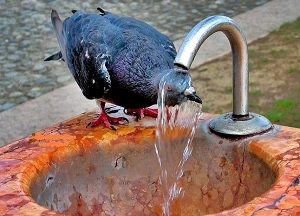How to write program in C for swapping two numbers without using third variable?Ricky 2 Points 1 Posts Hi, I am beginner in C and I'm stuck in a program to swap two numbers without using third variable. I know the program of swap two no's using temporary variable. Please, help me. Views: 8164 Total Answered: 2 Total Marked As Answer: 0 Posted On: 26-Jun-2016 01:28Kailash... Participant 66 Points 28 Posts Hello Ricky  Check this solution.  x=10; y=20;    x=x+y; -> x= 10+20=30y=x-y;  -> y= 30-20 =10x=x-y;  -> x=30-10=20; Posted On: 29-Jun-2016 08:54Smith Participant 374 Points 112 Posts Use following program in c: ``#include#includevoid main(){       int a=3,b=4;       clrscr();       printf("\n Before swapping :");       printf("\n a = %d  and  b = %d",a,b);       a=a+b;       b=a-b;       a=a-b;       printf("\n a = %d and b = %d",a,b);       getch();}`` Posted On: 06-May-2017 02:00